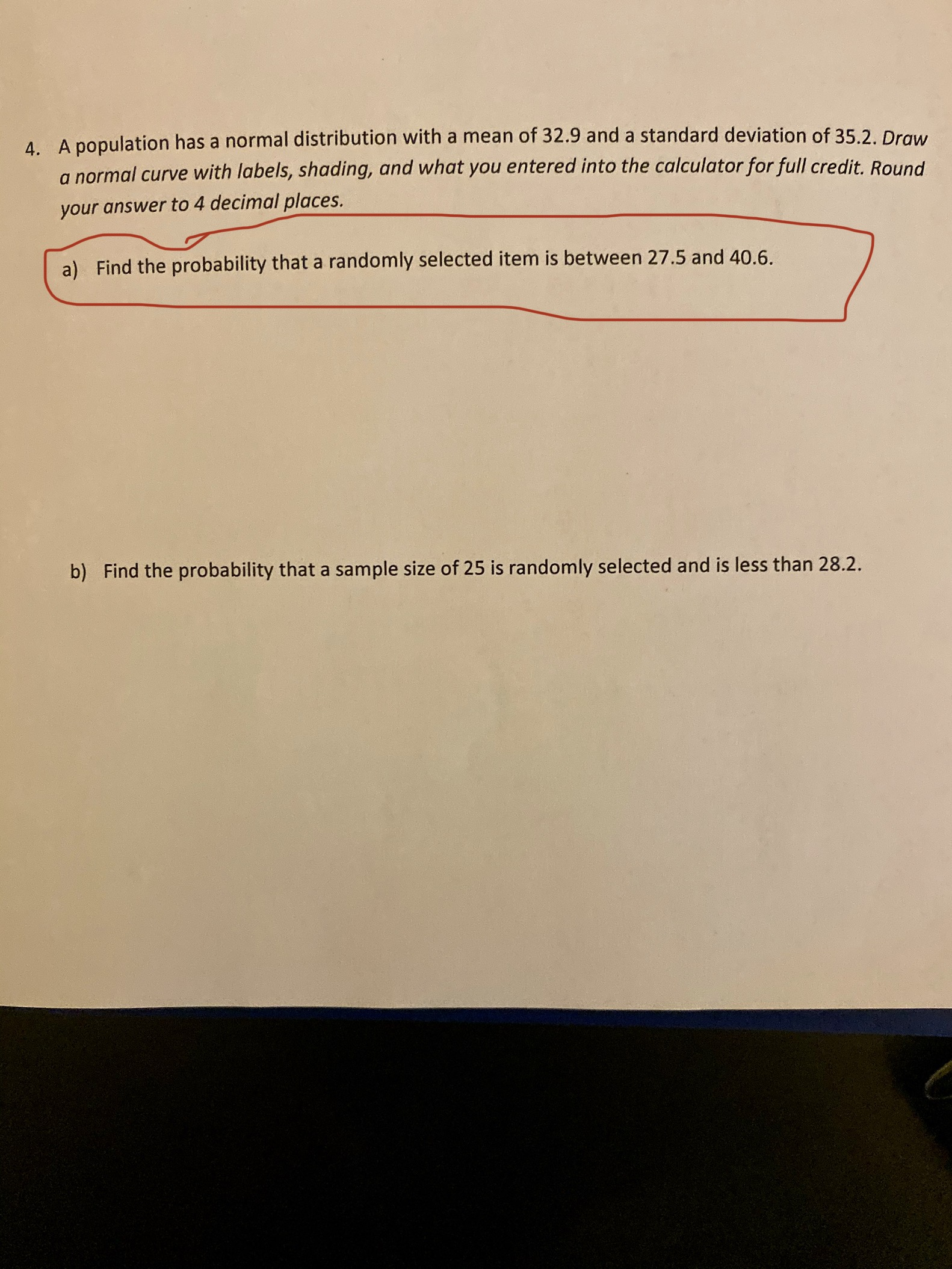# 4. A population has a normal distribution with a mean of 32.9 and a standard deviation of 35.2. Drawa normal curve with labels, shading, and what you entered into the calculator for full credit. Roundyour answer to 4 decimal places.Find the probability that a randomly selected item is between 27.5 and 40.6a)b) Find the probability that a sample size of 25 is randomly selected and is less than 28.2.

Questionhelp_outlineImage Transcriptionclose4. A population has a normal distribution with a mean of 32.9 and a standard deviation of 35.2. Draw a normal curve with labels, shading, and what you entered into the calculator for full credit. Round your answer to 4 decimal places. Find the probability that a randomly selected item is between 27.5 and 40.6 a) b) Find the probability that a sample size of 25 is randomly selected and is less than 28.2. fullscreen
check_circle

From the given information mean and stan...

### Want to see the full answer?

See Solution

#### Want to see this answer and more?

Solutions are written by subject experts who are available 24/7. Questions are typically answered within 1 hour.*

See Solution
*Response times may vary by subject and question.
Tagged in

### Probability